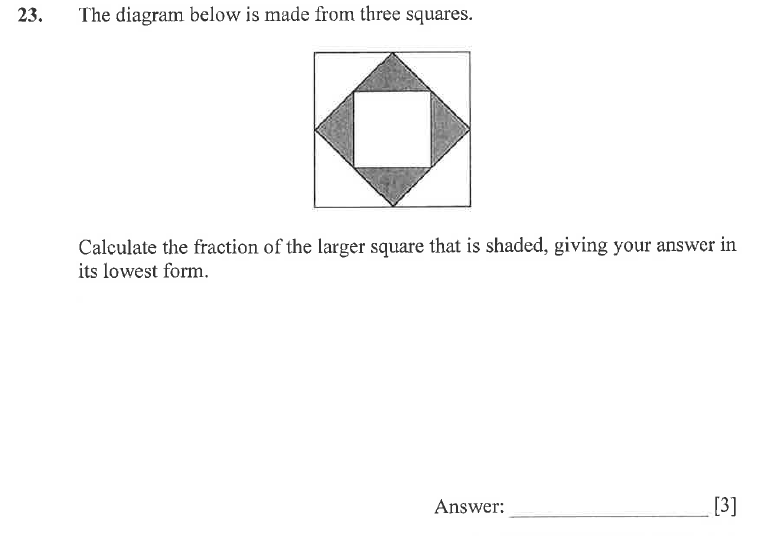# Dulwich College Maths Problem

July 31, 2015

How to solve the most difficult question on the Specimen A Maths Paper

The difficulty of 11+ maths papers generally progresses from simple numeracy problems at the beginning, to challenging logical reasoning and problem solving by the end. Dulwich College maths papers are no exception. Here's how to do the last problem on their specimen maths paper (A).

Here's the question:Note the  by the answer line tells you this question is worth 3 marks. When questions are worth more than 1 mark, it generally means some working out is required to get full marks. This also means you can get partial marks for correct working out, even if you don't get the correct answer!

Key words from the question: fraction, lowest form

Pay attention: They have asked for the fraction of the "larger square". This may throw some students; it tests student's understanding of the meaning of fractions rather than just rote memory of fraction processes.

How to solve it

In order to work out fractions of a large shape, the shape needs to be sliced into equal sizes. This can be done in many ways. See the image below. If you draw an "X" through the middle of the shape, you divide the shape into triangles which are all the same size as the shaded triangles.

Now count how many of these triangles you have total (16), and how many of them are shaded (4). Therefore, 4/16 are shaded. Now, as the question said "lowest form" you must reduce 4/16. Divide both the numerator (top) and denominator (bottom) of the fraction by 4. This will give  you 1/4.

Marks Scheme

1 mark for making any reasonable attempt at creating fractions.

1 mark for getting "4/16" (THIS MUST BE WRITTEN ON THE PAGE!)

1 mark for correct answer "1/4"## Have you thought about online lessons?

Connect one-to-one with a tutor or share your lesson with a group for 50% of the tutor's hourly rate!

#### Notting Hill and Ealing School Past Paper (Maths)

A maths past exam from 2008 for Notting Hill and Ealing School, part of the London Independent …

#### 11+ English exercises for home

Here is a list of a few little exercises to follow at home to help with creative writing and …